We already stated the guiduing principle to SoulÃ©’s geometry over F_un; namely, that the notion of variety over the field with one element ought to be well bahaved under extension of scalars to the ring of integers.

We also mentioned the fancy Grothendiecksque definition of a scheme over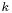as a (locally) representable functor from-algebras to sets. If you are one of the many people who are more used to think in geometrical terms rather that using a fanshi-wanshi categorical interpretation this might sound scary, but the idea behind it is actually quite simple: given a scheme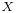over(defined in any classical way you feel comfortable with) we can associate to it the functorthat assigns to every (commutative) algebrathe set of morphisms of schemes between the spectrum ofand, which is what is normally called the set of-points of.This kind of construction is sort of standard in abstract algebraic geometry, and often receives the name of the functor of points. In the simplest case, when we are dealing with an affine scheme, i.e. something given as, then the-points ofare simply the ring homomorphisms fromto. Now, this gives us a functor associated to our scheme, but, is this a good correspondence? In other words, can we recover all the structure of the scheme just using the functor of points? The answer to this question is yes, we can, and the reason lies on a subtle but important fact: we have enough objects in the source category (i.e. enough commutative algebras) so that the scheme is fully determined by its points on every single algebra. For practical purposes, we might replace the category of all algebras by something smaller but still rich enough, and that would still give us an equivalent definition of scheme. This will come handy when we try to extend this definition to the case of varieties over. If all this sounds too complicated, you should maybe start by reading this other post first before trying to go on.

So, for SoulÃ©, a gadget (for lack of a better translation of the French ‘truc‘)Â  overconsists on a couple, where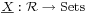is a (covariant) functor defined on a suitable categoryand landing on sets, andis a (complex) algebra. The functor should be the analogous to the functor of points given by a cassical variety; tricky point now is the choice of the category, which would be the substitute of the category of algebras. Different choices here will lead to different versions of geometries. If our category is too big we might be in trouble to find any examples of functors, and if it is too small it might happen that the functorial information is not enough to recover a geometrical object. There is no canonical choice here. Since our base test object are the field extensions of, the categoryshould contain at least the rings, and if we ever want to be able to define a nice cartesian product of varieties, we’d better drop in their tensor products as well. This would give us a minimal choice for our base category. A richer choice, which is indeed the one chosen by SoulÃ© in his paper is taking the categoryof all (commutative, unital) rings that are flat and finitely generated over the integers (recall that every ring is a module overin a canonical way). These conditions, on a ring, just mean that the underlying additive group ofis a lattice of finite rank. Examples of such kind of rings are for instance all the rings of integers of number fields, such like,and similars. The ringsare of course of this type, since they are the rings of integers of cyclotomic extensions of the rationals.

As we already mentioned, the choice for this category for defining gadgets can be pushed further. One possible refinement, undertaken by Connes and Consani, and explained here, is not taking rings but abelian groups instead. Since every ring is also an abelian group, this produces quite an enlargement of our testing category, and thus a way more restrictive notion of geometry.

But for now, we shall stick to our categoryof nice rings. So, as we were saying, a gadget is given by a functor, plus an algebra. We will also require that for any ring morphismand every element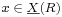we have a way of “evaluating” elements of the algebrain, like if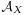were an algebra of functions (which will often be the case). More concretely, we will require the existence of algebra homomorphisms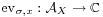, and that these evaluation maps behave well with respect to morphims in, meaning that whenever we have a morphism, for any point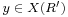we have the equality.

The rÃ´le of the algebrais a bit mysterious at the moment. The reason for introducing it is that, unlike in the classical case, the functor of points is not enough to determine the extension of scalars toof a hypothetical variety over. Morally, this algebra will represent a “given topology at infinity”, in the spirit of Arakelov geometry. The properties that this algebra ought to satisfy are not clear at the moment, so we will be quite vague about them.

Now that we have a collection of objects, our gadgets, we need an appropriate notion of morphism between gadgets. For gadgetsand, a morphismwill consist on a couple, whereis a natural transformation of functors, andis an algebra morphism such that, for each ring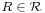,algebra map, and eachwe have the equalityas maps from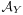to.

Composition of two morphismsandis simply given by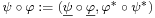.

All the above added up together, we have constructed a category, called the category of gadgets over. This is not yet what we need in order to have a variety with the properties we expect, so we will have to put some restrictions (related to the ‘local representability’ of the functor of points) in the definition… but we will save that for the next time!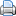Print This Post

1.